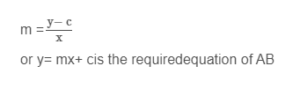# Co-ordinate Geometry

By Notes Vandar

Equation of straight line in slope intercepts form:Let P(x, y) be the any point line AB which makes a positive angle θ with x-axis.

From figure

∠ ACD= ∠ABE = θ

Slope of line AB = tanθ = m

PD = y- c

CD = OE =xAngle betweenlines y = m1x+ c2andy =mx+ c1Important Questions